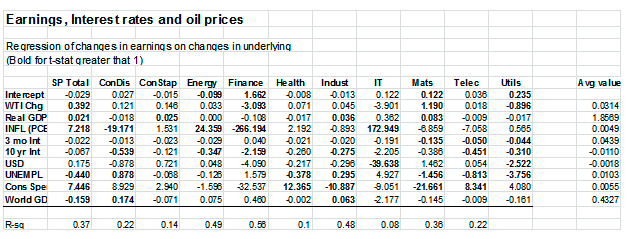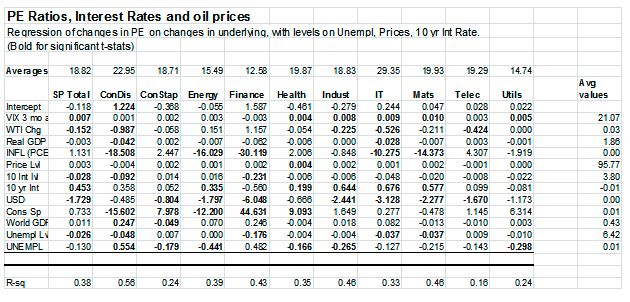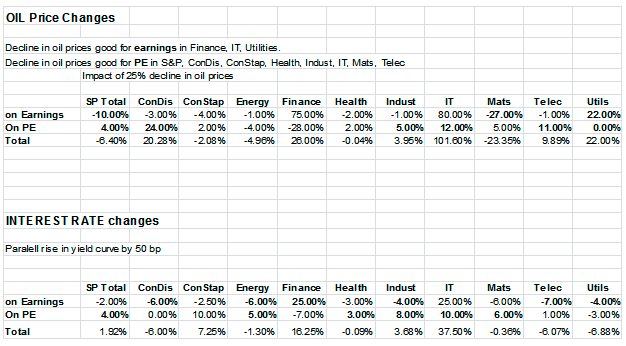# Estimating The Impact Of Oil Prices And Interest Rates On The S&P 500

by Eric Stubbs

Two important developments in 2014 will affect U.S. equity markets in 2015:  the precipitous decline in oil prices and increasing expectations that the Fed will begin raising short-term rates sooner rather than later.  But how specifically will these affect our stock market?

Below, I report on the results of an econometric model that I developed to address these questions.  Looking at 15 years of quarterly data, I estimated the impacts of a variety of factors on S&P 500 performance, including oil prices and interest rates.  I estimated impacts on earnings and price-earnings ratio.  The estimated impact on the S&P 500 level is the product of these two.

I conclude that a 25% decline in spot oil prices could reduce the S&P by 6.4% overall, but that the impacts will vary according to sector.  Overall impacts will be positive for consumer discretionary, financial services, information technologies (IT) and utilities.  They will be negative for materials, energy and consumer staples.

A 50 basis point (half-a-percent) parallel move up in interest rates would have a slight positive overall effect on the S&P 500, increasing it by 1.9%.  It would be most positive for IT, financial services and consumer staples.  Utilities, telecomm and consumer discretionary sectors would be negatively impacted.

Introduction

Two of the most significant stories of the last few months of 2014 for equity investors have been the declining price of oil and the anticipation that the Fed might start raising interest rates before the middle of 2015.

There’s no shortage of opinions about the effects that these developments might have on the U.S. equity market.  Oil price declines might, for instance, have several effects including:

• Increasing earnings in oil-using sectors
• Decreasing revenues and earnings in oil producing sectors
• Increasing consumer discretionary spending and therefore, in GDP growth
• Increasing the trade-weighted value of the U.S. dollar, reducing exports and increasing imports.

Likewise, rising interest rates may have a number of effects, differing for net borrowers versus savers and depending on whether the increases in short-term rates occur with no impact on the rest of the yield curve, or if the yield curve move is more or less parallel.

These are complicated questions and no solvable by either intuition or simple references to past events that might have been similar.  Consequently, I built a statistical model of drivers of the S&P 500 index to try to estimate these complex effects and assign directions and magnitudes to them.

Estimation strategy

To analyze these questions, I constructed a linear-regression model to uncover the impacts of various forces on the S&P, among them, oil prices and interest rates.

A macro-economic model of market behavior can involve extraordinary complexity.  One way to capture that complexity is through a multi-equation simultaneous structural model that attempts to quantify all of the intricate relationships among the variables of interest, as well as GDP, labor supply and demand, taxation, international trade, consumer spending, expectations, eventually culminating in a relationship that describes corporate earnings and their translation into a stock-market price level.  Such a model might also contain time leads and lags, and distinguish instantaneous impacts from longer term effects.

To develop such a model would be an ambitious undertaking and very demanding of the available data.  It is also one that may lead nowhere in terms of the ability to accurately model these many relationships, fit data to them and ultimately predict the effects of the variables of interest – oil prices and interest rates – on the stock market.

Instead, I took the approach of simplicity:  A single equation, reduced-form model.  Such a model simplifies the actual connections and relationships in an economy and stock market.  However, it is a good first approximation of the effects that we care about.  If a simple, linear model doesn’t pick up a statistical effect, it might well be that the effect is very weak or hasn’t been previously observed, or is counteracted by other confounding economic forces.  On the other hand, if a simple linear model finds an effect, it is probably there in some form, though not necessarily exactly as modeled.

The second consideration with any statistical estimation of market effects is the possibility that the scale of the change that we are seeking to understand is very different from any experienced in the sample period.  For instance if typical oil-price changes are in the order of, say, 5% per quarter and we estimate a model seeking to understand the impact of a change of 25% per quarter, that will take us into uncharted territory.   A linear model can exacerbate this problem.  If a 5% change in oil prices causes a 3% change in earnings, for example, it may be wrong to extrapolate to the conclusion that a 25% oil price change will necessarily have a 15% impact.  It could be more or less.  However, a linear model assumes that the effect is linear.

Those cautions notwithstanding, I constructed a simple linear model to try to capture the effects of the variables of interest on the S&P 500.  I estimated the model for the overall index as well as for the S&P sectors.

The level of the S&P 500 index, or any index, can be separated into the product of earnings (E) and the price-earnings ratio (P/E).

I estimated equations for each of these.  I modeled earnings to depend on oil prices, real GDP, inflation, three-month and 10-year interest rates, the value of the dollar, the unemployment rate, consumer spending and global GDP growth.  My P/E ratio model depended on the VIX (S&P volatility), oil prices, real GDP, the inflation rate, the price level, the level of 10-year interest rates as well as the change in that rate, the value of the dollar, consumer spending, global GDP, and the level and change in the unemployment rate.

I used 15 years of quarterly data ending with the second quarter of 2014 to estimate the equations. The equations are shown in the appendix.

Results

The table below summarizes the regression results for the impact of various macro-economic variables on S&P earnings.  I ran the regression in fractional change terms so the coefficients represent elasticities (fractional change in earnings per unit fractional change in the explanatory variable.)

For the most part, the results for the S&P 500 (SP Total) correspond to expectations.  Real GDP change has a positive effect.  Inflation change has a strong positive effect, as does consumer spending.  An increase in the value of the dollar has a negative effect – perhaps due to the negative impact on the demand for exports.  Interest rates also have a negative effect.  Rising oil prices (WTI chg) have a positive effect on earnings (and falling oil prices – the case that we are trying to understand – has a negative effect on earnings.).  Also a change in the unemployment rate has a negative effect.  That is, rising unemployment reduces earnings.  Perhaps the only counterintuitive result is the negative coefficient on world GDP growth – indicating that S&P earnings decline with rising world GDP.Most of the sector-specific results followed expectations with a few conspicuous exceptions.  Rising (falling) oil prices have a negative (positive) effect on earnings in financial services, IT and utilities.  A parallel upward shift in interest rates generally has a negative effect on earnings, but because the implied shift would be proportionately greater at the short end of the yield curve (meaning that the yield curve flattens), the net impact on financial services and IT are positive.  Real GDP growth has a negative impact for a few sectors – consumer discretionary, finance, health, telecomm and utilities – but this effect is nearly zero and not statistically significant in any of these cases.  Unemployment growth is also generally negative in most cases.  It is positive and significant only for consumer discretionary and industrials.  Consumer spending also generally follows intuitions.   It is negative and significant only for industrials and materials.  Since these are largely non-consumer sectors, it stands to reason that for a given level of GDP, greater consumer spending (lower corporate spending) would have a negative impact on earnings in these sectors.

Overall, the IT and financial services regressions are the least reliable of these estimations.

Turning now to the P/E ratio regressions, those results appear in the table below.  Here I ran the regression on a combination of changes and levels, complicating to some degree the determination of elasticities as they will vary with levels.

Looking first at the S&P Total results, I found most of the results follow expectations.  One interesting result is that the VIX – a measure of market volatility – doesn’t appear with the expected negative sign.  Moreover, contrary to expectations, its influence throughout is trivial.  Oil price increases (decreases) have a negative (positive) impact on market P/Es.  The short-term rate had no discernible impact and I dropped it from the regressions.  The impact of a rising 10-year rate was net positive for P/Es overall and in most sectors except financial services and utilities.  Inflation increases have a small positive impact overall on P/Es although it is a negative influence on P/Es for consumer discretionary, energy, financial services, IT and materials.  Consumer spending has a positive impact on overall P/Es although it is negative for some sectors.  Increases in the value of the dollar have a generally negative impact on P/Es.  Finally, the unemployment level is generally a negative influence on P/Es as is rising unemployment.Conclusions

We can summarize these results in a table of oil price change impacts and interest rate change impacts on Earnings and PEs, leading to an overall impact on the market level.  I show those results below for the S&P 500 as a whole as well as by sector.Overall, I found that a 25% drop in oil prices might cause the S&P 500 to decline by 6.4% — due to a 10% decline in earnings and 4% increase in P/Es.  According to these estimates, the energy and materials sectors would suffer the largest declines.  The sectors most advantaged would be consumer discretionary, utilities, financial services and IT – although I do not consider the estimations for the last two to be reliable.

I estimated the impact of a 50 basis point parallel shift up in the yield curve to be a small net positive for the S&P 500 (+1.9%).  It is especially positive for financial services, IT and consumer staples, with the same caveats applying to the first two.  It is estimated to be a negative for utilities, telecomm and consumer discretionary sectors.

It is helpful to look at the estimated impacts of some of the other variables to get a sense of how these effects compare to others in the economy. A 4% rise in GDP for instance, is estimated to have a +8% impact on the S&P – mostly through earnings improvements.  A 0.2% drop in the unemployment rate has an estimated +10% impact on the S&P, also primarily through earnings.  A 3% rise in consumer spending has an estimated +20% impact on the S&P.

Appendix:  Explanation of the statistical equations and variables

Starting with the S&P price level (P), I decomposed that into the product of earnings (E) and price-earnings ratio (P/E).

P = E * PE       (1)

I then modeled E and P/E as linear functions of various economic variables:

E = a + b1(WTI) + b2(Real GDP) + b3(INFL) + b4(3 mo. INT) + b5(10 yr INT) + b6(USD) + b7(UNEMPL) + b8(Con Sp) = b9(World GDP)                (2)

WTI = WTI Spot oil price
Real GDP = Real US GDP
INFL = Inflation (CPI)
3 mo. INT = Three-month Treasury interest rate
10 yr INT = Ten-year Treasury interest rate
USD = Trade weighted value index for US dollar
UNEMPL = U3 unemployment rate
Con Sp = Consumer spending (GDP component)
World GDP = Global GDP

I estimated this equation, like the P/E equation below, as a percentage change in quarter-over-quarter, not as a level.  This is a simple way to de-trend data for estimation purposes.  It provides a better test of whether an effect is real or not, as the regression gets little benefit from the typical correlation in upward trending data series that one frequently sees in economic and market data.   It also allows for a more straightforward comparison over time since magnitudes of effects become less susceptible to inflationary growth with the passage of time.

P/E Ratio:

PE = a + b1(VIX) + b2(WTI) = b3(Real GDP) + b4(INFL) + b5(Price Lvl) + b6(10 INT Lvl) + b7(10 INT chg) + b8(USD) + b9(Cons Sp) + b10(World GDP) + b11(UNEMPL lvl) + b12(UNEMPL chg)                                (3)

For the PE equation, I used several of the variables from the E equation (2), with a few additions.

Price Lvl = Level of the price index
10 INT Lvl = Level of nominal 10-year Treasury rate.
UNEMPL Lvl = Level of the U3 unemployment rate.